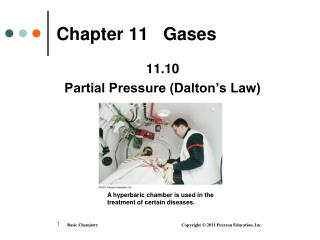Download PresentationChapter 11 Gases

Loading in 2 Seconds...

# Chapter 11 Gases - PowerPoint PPT Presentation

A hyperbaric chamber is used in the treatment of certain diseases. Chapter 11 Gases. 11.10 Partial Pressure (Dalton’s Law). Partial Pressure. The partial pressure of a gas is the pressure of each gas in a mixture is the pressure that gas would exert if it were by itself in the container.I am the owner, or an agent authorized to act on behalf of the owner, of the copyrighted work described.
Download Presentation## Chapter 11 Gases

An Image/Link below is provided (as is) to download presentation

Download Policy: Content on the Website is provided to you AS IS for your information and personal use and may not be sold / licensed / shared on other websites without getting consent from its author.While downloading, if for some reason you are not able to download a presentation, the publisher may have deleted the file from their server.

- - - - - - - - - - - - - - - - - - - - - - - - - - E N D - - - - - - - - - - - - - - - - - - - - - - - - - -
Presentation Transcript
1. A hyperbaric chamber is used in the treatment of certain diseases. Chapter 11 Gases 11.10 Partial Pressure (Dalton’s Law)

2. Partial Pressure The partial pressure of a gas • is the pressure of each gas in a mixture • is the pressure that gas would exert if it were by itself in the container

3. Dalton’s Law of Partial Pressures Dalton’s law of partial pressuresstates that the total pressure • depends on the total number of gas particles, not on the types of particles • exerted by a gas mixture is the sum of the partial pressures of those gases PT = P1 + P2 + P3 .....

4. The total pressure of two gases is the sum of their partial pressures. Illustrating Partial Pressures

5. Under water, the pressure on a diver is greater than the atmospheric pressure. Scuba Diving • When a scuba diver is below the ocean surface, the increased pressure causes more N2(g)to dissolve in the blood. • If a diver rises too fast, the dissolved N2 can form bubbles in the blood, a dangerous and painful condition called "the bends.” • For deep descents, helium, which does not dissolve in the blood, is added to O2.

6. Solving for Partial Pressure

7. Learning Check A scuba tank contains O2 with a pressure of 0.450 atm and He at 855 mmHg. What is the total pressure in mmHg in the tank?

8. Solution STEP 1 Write the equation for the sum of partial pressures. Ptotal = Po2 + PHe STEP 2Solve for the unknown pressure. Convert the pressure in atm to mmHg to match units. 0.450 atm x 760 mmHg = 342 mmHg = PO2 1 atm STEP 3Substitute known pressure and calculate the unknown. Ptotal = 342 mmHg + 855 mmHg = 1197 mmHg

9. A Nitrox mixture is used to fill scuba tanks. Learning Check For some dives, scuba divers use a mixture of nitrogen and oxygen gases (Nitrox) with a pressure of 8.00 atm. If the oxygen in a tank of Nitrox has a partial pressure of 2190 mmHg, what is the partial pressure of the nitrogen? 1) 520 mmHg 2) 2040 mmHg 3) 3890 mmHg

10. Solution STEP 1 Write the equation for the sum of partial pressures. Ptotal = PO2 + PN2 Ptotal = 8.00 atm x 760 mmHg = 6080 mmHg 1 atm STEP 2Solve for the unknown pressure. Ptotal = PO + PN2 2 PN2= Ptotal - PO2

11. Solution (continued) STEP 3Substitute known pressure and calculate the unknown. PN2= Ptotal - PO2 PN2= 6080 mmHg - 2190 mmHg = 3890 mmHg (3)

12. Gases Collected over Water A gas produced in the laboratory • usually contains water vapor • PT = Pwater + Pgas • has a partial pressure obtained by subtracting the vapor pressure of water from the PT

13. Gases Collected over Water

14. Learning Check The decomposition of KClO3 produces O2 gas and solid KCl. If 124 mL of O2 is collected over water at 762 mmHg at 20.0 C, how many moles of O2 are produced? (Partial pressure water at 20.0 C = 18 mmHg.) 2KClO3(s) 2KCl(s) + 3O2(g)

15. Solution STEP 1 Obtain the vapor pressure of water. Partial pressure of water at 20.0 C = 18 mmHg STEP 2Subtract vapor pressure from total P of gas mixture to give partial pressure of needed gas. PT = Pwater + Pgas Pgas = PT - Pwater PO2 = 762 mmHg - 18 mmHg = 744 mmHg

16. Solution (continued) STEP 3 Use ideal gas law to convert Pgasto moles or grams of gas collected. PV = nRT n = PV = (744 mmHg)(0.124 L) = 0.00505 mol of O2 RT (62.4 L • mmHg)(293 K) mol • K

17. Gases We Breathe The air we breathe • is a gas mixture • contains mostly N2 and O2 and small amounts of other gases

18. Gas Exchange During Breathing

19. Blood Gases • In the lungs, O2 enters the blood, while CO2 from the blood is released. • In the tissues, O2 enters the cells, while CO2 is released into the blood.

20. Blood Gases In the body, • O2 flows into the tissues because the partial pressure of O2 is higher in the blood and lower in the tissues • CO2 flows out of the tissues because the partial pressure of CO2 is higher in the tissues and lower in the blood